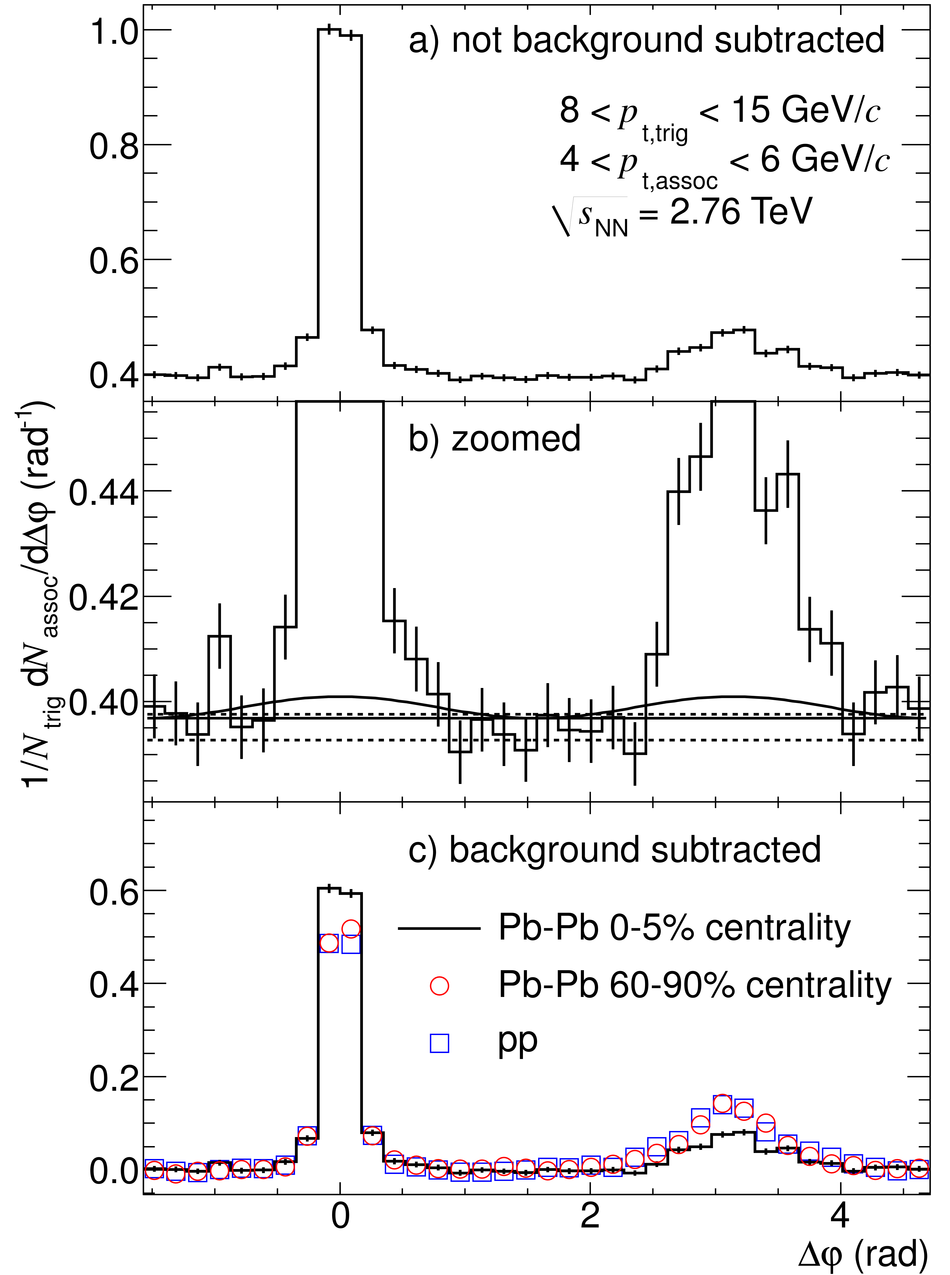# Particle-yield modification in jet-like azimuthal di-hadron correlations in Pb-Pb collisions at $\sqrt{s_{\rm NN}}$ = 2.76 TeV

The yield of charged particles associated with high-$p_{\rm T}$ trigger particles ($8 <~ p_{\rm T} <~ 15$ GeV/$c$) is measured with the ALICE detector in Pb-Pb collisions at $\sqrt{s_{\rm NN}}$ = 2.76 TeV relative to proton-proton collisions at the same energy. The conditional per-trigger yields are extracted from the narrow jet-like correlation peaks in azimuthal di-hadron correlations. In the 5% most central collisions, we observe that the yield of associated charged particles with transverse momenta $p_{\rm T}> 3$ GeV/$c$ on the away-side drops to about 60% of that observed in pp collisions, while on the near-side a moderate enhancement of 20-30% is found.

Figures

## Figure 1

 Corrected per-trigger pair yield for $4 < {p_{\rm t, assoc}} < 6GeV/c$ for central Pb--Pb events (histogram), peripheral Pb--Pb events (red circles) and pp events (blue squares) a) azimuthal correlation; b) zoom on the region where the pedestal values (horizontal lines) and the $v_2$ component ($\cos 2 {\Delta\varphi}$) are indicated. Solid lines are used in the yield extraction while the dashed lines are used for the estimation of the uncertainty of the pedestal calculation; c) background-subtracted distributions using the flat pedestal Error bars indicate statistical uncertainties only.## Figure 2

 a) $I_{\rm AA}$ for central (0-5% Pb--Pb/pp, open black symbols) and peripheral (60-90\% Pb--Pb/pp, filled red symbols) collisions.b) $I_{\rm CP}$. Results using different background subtraction schemes are presented: using a flat pedestal (squares), using $v_2$ subtraction (diamonds) and subtracting the large $|\Deta|$-region (circles, only on the near-side). For details see text. For clarity, the data points are slightly displaced on the $p_{\rm t, assoc}$-axis. The shaded bands denote systematic uncertainties.}